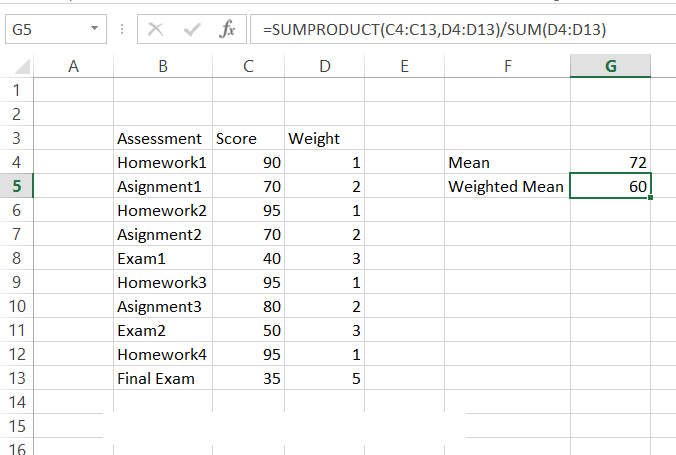### How To Calculate Weighted Average In Excel

In this Excel lesson you will teach yourself what is weighted average, how and when to use it. Also you will see many examples how to handle weighted average in Excel.

## What is weighted average?

Weighted average is (next to arithmetic mean) one of the most common concepts in math lessons. Just like the name suggests, it is used to obtain the average of a specific group of numbers. However, it differs from the mean in that each number has a specific weight.

In other words, the individual numbers are more or less important and thus have an impact on the final result for others. What determines the importance of a number is the weight assigned to it.

## When to use weighted average?

Weighted average can be used in a variety of situations. It is most commonly found in the school system, where it is used to calculate semi-annual or end-of-year grades. This is because the scores may have a different value.

It is clear that a quiz test spanning several lessons should have less impact on the end-of-year assessment than a serious test covering a wide range of knowledge. As a result, teachers often give a specific weight to scores.

It should be remembered, however, that the weighted average also does not remove subjectivism from the teacher’s assessment. Ultimately, teacher is forced to assign a weight rating based on his individual feelings.

In practice, the weighted average may completely change the year-end rating. In extreme situations, depending on whether an arithmetic mean or a weighted mean is used, the difference in the year-end assessment may differ significantly.

Suppose our weighting set has n items.

Score 1 Weight 1

Score 2 Weight 2

Score 3 Weight 3

Score n Weight n

We will calculate the weighted average according to the following formula

(RATING1 * WEIGHT1) + (RATING2 * WEIGHT2) + (RATING3 * WEIGHT3) + (RATING * WEIGHT) / (WEIGHT1 + WEIGHT2 + WEIGHT3 + WEIGHT)

A weighted average can be used to evaluate different products. For example, if you run a website that deals with mobile phones, you can rank these devices. If you decide which parameters are more important than the others, you can give them more weight. Thanks to this, they will have a greater impact on the final grade.

One of the most famous stock exchange indices is the S&P 500, the value of which is determined on the basis of the weighted average capitalization of five hundred largest American companies.

## Weighted Average Formula

This is how to calculate weighted mean. Weighted average of numbers x1, x2, x3, …, xn with weights respectively w1, w2, w3, …, wn is given by the formula:

xw = (x1⋅w1 + x2⋅w2 + x3⋅w3 + … + xn⋅wn) / (w1 + w2 + w3 + … + wn)`Weights w1, w2, w3, ..., wn should be non-negative numbers.`

You just need to add up all the scores multiplied by their weights, and then divide the result by the sum of all the weights.

## How to calculate weighted average in Excel

To calculate weighted average in Excel you need to know SUMPRODUCT and SUM Excel functions.

Weighted average Excel formula is:

=SUMPRODUCT(weights,values)/SUM(weights)

Sumproduct function is adding multiplies of scores and their weights. Then you need to divide it by sum of grades calculated by Excel SUM function.

## Examples of weighted average

### Example 1 simplified calculation of the survey

Group of people were asked how long they had slept during the last night.

=SUMPRODUCT(B4:B8,C4:C8)/SUM(C4:C8)It can be seen that this way of understanding the weighted average is like a “simplification” of the arithmetic mean. We could list all the observations and then calculate the arithmetic mean. As a result, we would get the same result. Such a procedure is used when we have predetermined (a finite number, in practice a small) cases and we know their number (how many have we recorded).

### Example 2 calculate weighted average of grades

Here’s another example to calculate weighted mean of school scores. I used the formula:

=SUMPRODUCT(C4:C13,D4:D13)/SUM(D4:D13)You may notice that weighted mean is much lower than mean. It happened because weight of exams is much bigger. Students results of exams and especially final exam is much worse than other scores.

### Example 3 portfolio rate of return

I need to calculate weighted average rate of return of portfolio.

One of the investment is much bigger than the others. Also rate of return of investment differs significantly.First I summed Value of investments using =SUM(C3:C7)

Next calculated weighted average with =SUMPRODUCT(C3:C7/\$C\$8,D3:D7)

The same I could do step by step.

First to calculate investment weights weights which are just Value / Total Value (=C3/\$C\$8)

Next check contribution of each investment multiplying each rate of return by weight (=D3*E3)

Weighted average is just sum of calculated contributions (=SUM(F3:F7)

## Template

```Further reading: